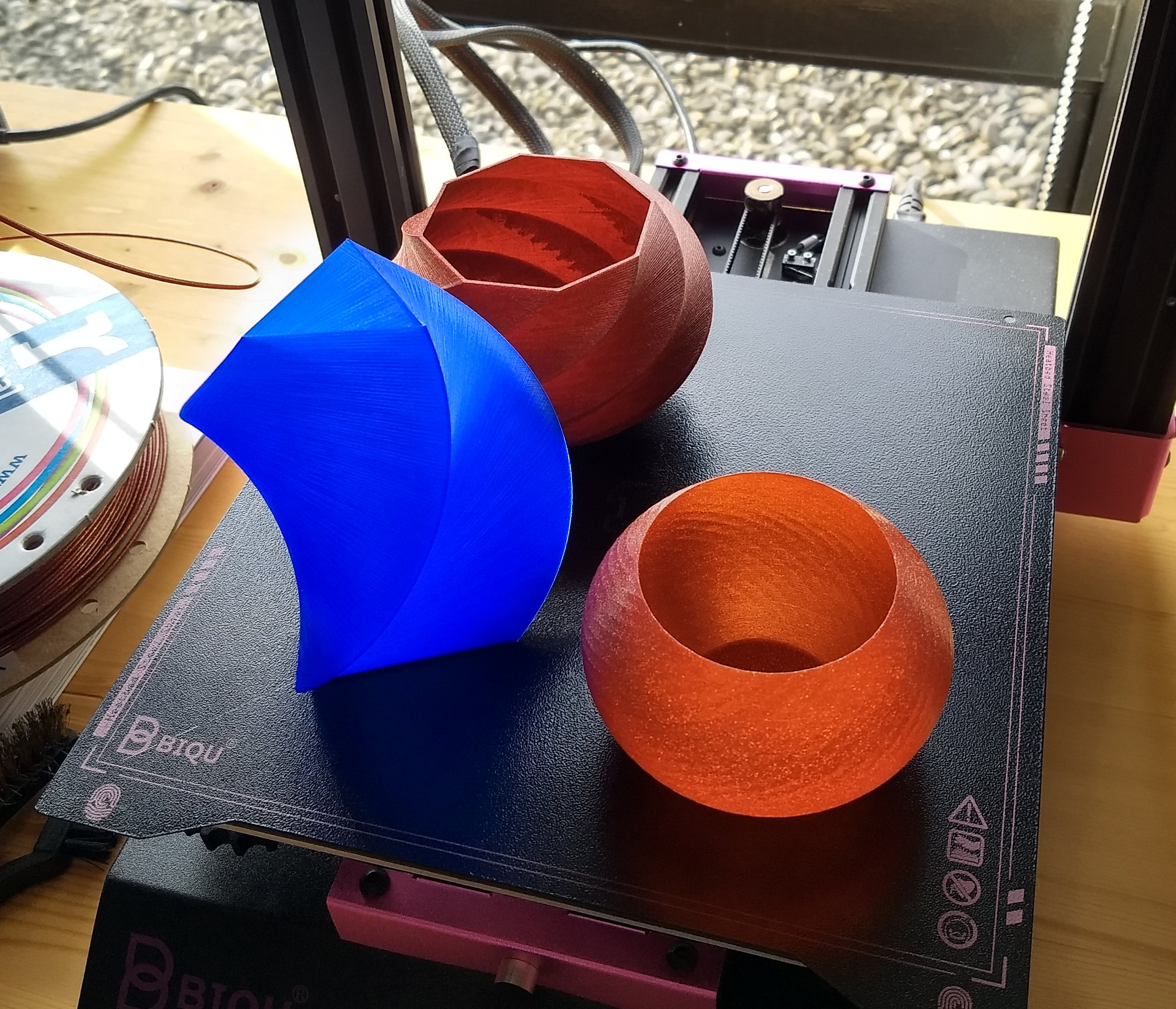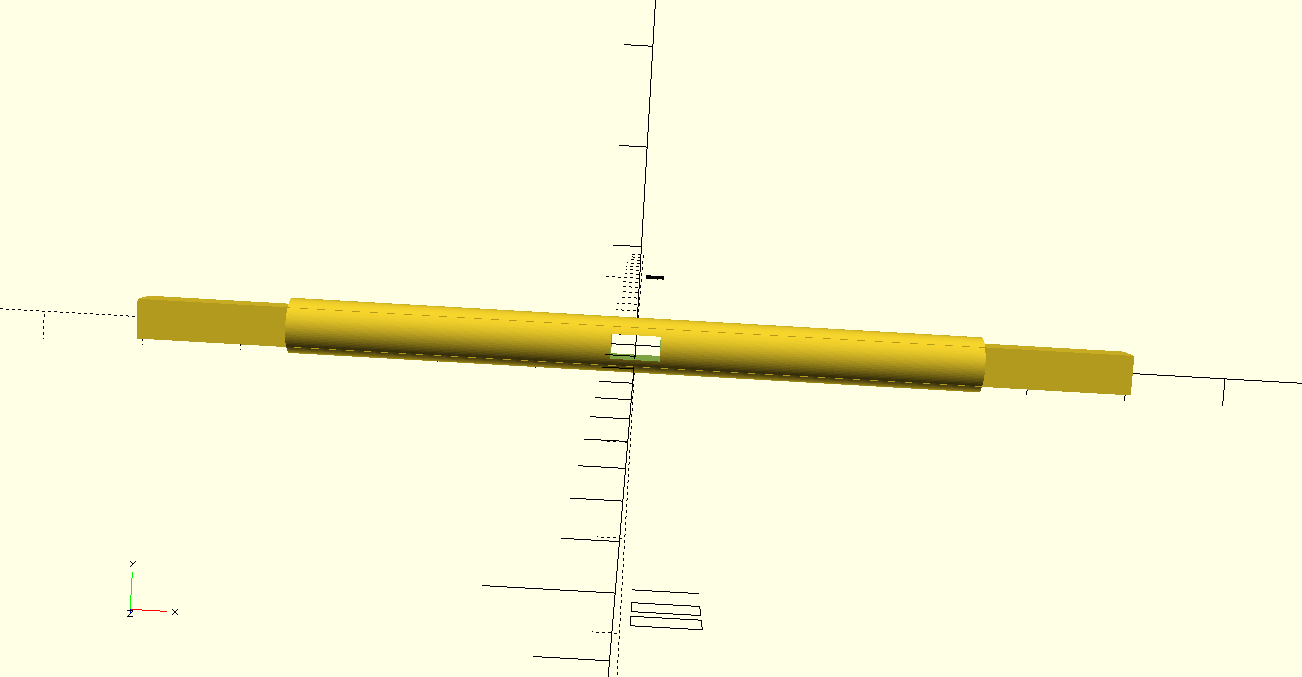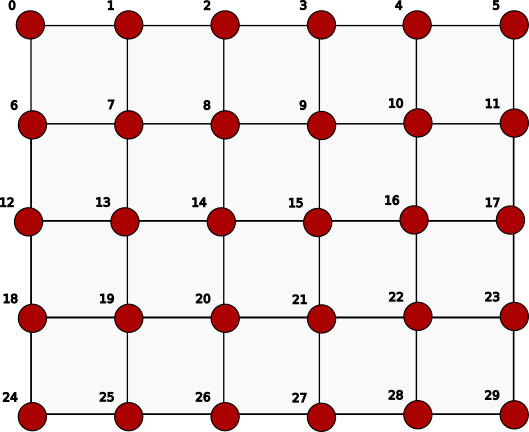Categories

Earlier I explained how to use polyhedron to create a regular cylinder in OpenSCAD. Not very exciting but this method is very useful to create much more complex objects. As long as we’re able to find the right function we can create the object with this method.

As an example I’ll explain how to create a twisted or spiraled vase. You’ve probably seen them on Printables or Thingiverse and they exist in many varieties. In this post we first create one starting with a simple triangle and then other regular polygons.Three examples of twisted vases created with this code. On the left in the front a twisted triangle (in blue), on the left in the back a twisted octagon and on the right a twisted regular polygon that consists if 36 edges or vertices.

For that we use the same method as in the previous post about polyhedron: first we calculate the points of the vase and then we define the faces.

But first consider a regular triangular plane and extrude that in the height (z-direction). Now suppose we twist this triangular plane while extruding and to make it even more complex increase or decrease the size of the plane while extruding. How would that function look like. In this example I’ll use a sinusoid or sine wave function. A sinusoid is the representation of any mathematical sine (or cosine) function that is smooth periodic in nature. Here is the general formula.

``f(z) = a + b * sin(c(z)+d);``

By substituting values for a, b, c and d we get very different sine functions. In this example I’ll use:

``f(z) = 2 + 2 * sin(z)``

Before we’re able to calculate our points we need the following variables.

``````height = 160; //total height of the vase
step = 1; //steps in z-direction. The lower the more faces
twist = 1.2; //increase to have more twist``````

The height is in the z-direction, the radius is the radius of the initial circle when all the three vertices of the regular triangle lie on that circle. Step is the step size in the z-direction and twist is the amount of twist that the vase gets. The higher is more twist. Then we need three angles to calculate the exact point of each triangle.

``sh3 = [0,120,240]; //triangle``

Now we can calculate all the points with the code below

``p = [for (z = [0:step:height], angle = sh3) [radius*cos(angle+twist*z)*f(z),radius*sin(angle+twist*z)*f(z),z]];``

It’s a nested loop where in the inner loop the angle is varied according to the values of sh3 and in the outer loop the value of z is varied. Hopefully you’ll see similarities the code below that we used in the earlier post to calculate the basic cylinder: `[cos(angle) * r, sin(angle) * r, z]`.

The first two values in the inner square brackets define the position of a point in the xy-plane. Notice that compared with the our earlier formula we multiply x and y values within the brackets with our sine function f(z). We also changed the parameter of the sine and cosine by adding the twist variable and multiply in with z.

Lastly, like we did with the cylinder, we have to create the faces. The code is similar to the code we used with the cylinder but with some essential changes.

``````module shape(p,m,n) {

fcs = [for (j = [1:m], i = [0:n-2])[(n*j)+i,(n*j)+1+i,(n*(j-1))+i+1,(n*(j-1))+i]];

top = [for (i = [n*m:(n*m)+n-1]) i]; //connect points to create the top face

bottom = [for (i = [0:n-1]) i]; //connect points to create the bottom

stitches = [for (j = [0:m-1]) [j*n,((j+1)*n)-1,(((j+2)*n)-1),(j+1)*n]]; //connect points between the first and the last face per row

//now connect all
//reverse is used to reverse normal of top face
fcsc = concat([bottom],fcs,stitches,[reverse(top)]); //concatenate the bottom, body and top

color("teal") polyhedron(points=p, faces=fcsc);
}``````

The module shape takes as parameters a list of points (p) and the rows and columns of a matrix (m * n). The matrix is calculated as follows.

``````m = floor(height/step); //numbers of rows of the matrix
n = len(sh3); //number of columns in the matrix``````

## Correcting the normals

Getting back to the shape module you’ll may be noticing that it differs from the cylinder version. One difference is can be found in the fcs list variable. When we watch closely we’ll notice that the for-loop for i has been reversed. This has been done to avoid errors in the stl file. As it turns out the normals of each face from fcs were pointing inwards into the object. OpenSCAD doesn’t complain about it but other programs like Prusaslicer do (see image below).Prusaslicer auto-repaired 2240 errors in this stl file that was exported from OpenSCAD. These errors are all inward pointing normals that need to be reversed to point outward.

The top also prompted errors and this can be solved by reversing the order of the top list. To do this we need another function called reverse. It reverses the order of the list that is provided as a parameter to it.

``function reverse(lt) = [for(i = len(lt) - 1; i > -1; i = i - 1) lt[i]];``

## Finishing the code

And this leaves only the last part of the code which is simply

``shape(p,m,n);``

The code that I’ll include with this post is a little more complicated. I added a Customizer to change some of the variables and I added a different sine function (g(z)) that provides a very different vase. I also added the angles for a pentagon, hexagon and octagon (sh5, sh6 and sh8) if you want to change the regular triangle for another regular polygon. If you do make sure to change sh3 on two places in the code: the value for the variable n and in the formula to calculate all points (p).

The code can be found in this link: https://eroic42.nohost.me/nextcloud/s/mMbtCciBMA7BEpj.

Categories

## A curved 3d printed lithophane in OpenSCAD

How can I wrap a flat surface around a cylinder in OpenSCAD. This was a question I asked myself. OpenSCAD doesn’t provide a ready made method so we need to write our own. Luckily we don’t have to invent the wheel here, others have done that already. Justin Lin has written a very handy tutorial on his website Openhome.cc how to wrap a text around a cylinder.  In this project I took it a step further and wrapped a png image over part of a cylinder resulting in a curved image.

It seemed like fun to combine this technique of wrapping with the 3D print of a lithophane. A lithophane is an engraved image on translucent material. Many 3D printers have already produced lithophanes sometimes with stunning results. For this experiment I’ll use white PLA because it  has excellent translucent properties.

Now first let me explain the basics on how to wrap a 2D image around a cylinder. First we create a circle out of triangles. These triangles are then extruded to segments to form a cylinder.  Next all segments of the cylinder are lined up in a row and the image is place on the far side but just within the limits of the segments. When we intersect the image with every individual segment and return the segments to their original position in the cylinder, a curved image emerges (see images below).

If you want to know more please watch my video below. In this video the OpenSCAD script is explained and demonstrated. Also is shown how to import the stl file in Cura and what settings are used.

Here you can download with an example file of a curved lithophane:

If you want to use your own png file change the surface command in the OpenSCAD file to include your own png file. Make sure to also change the height and width according to the imported png image in px. Prerender and render can take some time so it’s recommended to use a fast PC. And the larger the png the longer it takes.

Categories

## Peddle wheel boat (3D printed)

I found an example on Thingiverse, a rubber band powered boat with two peddle wheels, but it has two problems. First of all the author only provides .stl files and second the design is a bit flawed. I therefore decided to design the boat from scratch with the 3d CAD program OpenSCAD. With OpenSCAD I’m not only able to edit my models quickly, I’m also able to share the OpenSCAD script allowing other to use and change it. Links to the downloadable files can be found here: https://my.hidrive.com/share/pyilt3itb8

The Paddle Wheel Boat that I created consists of four parts: the boat, the shaft and two peddles. The main change of the boat that I designed is the position of the shaft. It’s been shifted to the middle of the boat providing more balance. I also enforced the attachment point of the rubber band to the boat making it impossible to break it.

I also increased the size of the paddles enabling the boat to move faster. The shape of the paddles are rounded instead of square improving the dynamics of the paddles through the water. A problem with the old design is that the rubber band has to be fixed to the shaft with some tape. Not a very elegant solution. I added a square hole to the design of the shaft. The rubber band can be inserted through the hole and fixed to the shaft. No tape needed.Simple square hole in the shaft. The rubber band fits right through the hole making it easy to fix it to the shaft.

Changes to the models are easily made. The OpenSCAD script is simple and the result of a change can be reviewed immediately by pressing F5. E.g to move the shaft to the back of the boat can be achieved by just one simple change in the script.

Just four parts, the boat, shaft and two peddles, are needed to make the boat. If you don’t want to make changes to the design just download the provided .stl files and open them in your favourite slicer. After printing the Peddle wheel boat can be assembled and tested. Have fun.

Categories

Writing a script for a simple hook in OpenSCAD is easy but I wanted to do something more and make a parametric hook. With parametric I mean that a user can easily adjust the script by changing some variables to make your own hook.

To make it even easier the script makes use of the Customizer of OpenSCAD. This means that users don’t have to tinker with the code but can adjust the values of the variables with a easy to use panel on the right side of the GUI of OpenSCAD called the Customizer. (If you don’t see the Customizer in OpenSCAD go to the menu bar and click on Windows and then Customizer).

Aside from changing the dimensions I also added the possibility to add a fillet or a chamfer to the hook. And I added the option to change the radius of the hook and the diameter of the screw holes.

If you’re interested here is an explanation of some parts of the code. The fillet of the cube shaped parts of the hook is created with the fillet module. Within this module I simply intersect a cylinder with radius r1 and a cube of the desired length l.

``````module fillet(l,r1) {
intersection() {
cube([l,l,l]);
cylinder(h=l, r=r1, \$fn=50);
}
}``````

In the module roundedCube the thus created fillet pieces are positioned in a such a way that the hull command shapes them to the desired filleted cube.

``````module roundedCube(l,w,h,r1) {
d = 2 * r1;
translate([r1,r1,0])
hull() {
rotate([0,0,180]) fillet(l,r1);
translate([0,w-d,0]) rotate([0,0,90]) fillet(l,r1);
translate([h-d,0,0]) rotate([0,0,270]) fillet(l,r1);
translate([h-d,w-d,0]) rotate([0,0,0]) fillet(l,r1);
}
}``````

https://www.printables.com/model/91734-parametric-hook-with-source-file

EDIT: V1.1 of the OpenSCAD file (.scad) has the option to add a countersunk to the screw holes.

Categories

OpenSCAD allows the user to create complex shapes with the polygon function for 2D and polyhedron for 3D. Polygon and polyhedron both accept a list of 2D and 3D coordinates (points) respectively as parameters. A functions can generate a list of points eliminating the need to manually created these lists. This property can be used to create shapes that are impossible with the 2D and 3D shapes that are build-in in OpenSCAD. In this blog post I’ll show how to create some simple 2D and 3D shapes and explain how to create more complex shapes.

## Creating a 2D shape

To create a circle with a radius of 20 in OpenSCAD we just have to type

``circle(20);``

However OpenSCAD doesn’t allow us to reshape this build-in function to for instance an ellipse. Alternatively we can write a function that generates a list of points needed for a circle and then use polygon with the points as parameter to draw the circle. The function uses the trigonometric formulas, x = r cos φ and y = sin φ, to convert polar coordinates to Cartesian coordinates.

``````function circle(radius) = [for (phi = [1 : 1 : 360]) [radius * cos(phi), radius * sin(phi)]];
polygon(circle(20));``````

When F5 is pressed a circle is drawn however the x,y coordinates of this circle are available to us. By adding echo(circle(20)); to our script the list of points is printed in the console. The circle function can easily be altered thus gaining a new shape. An example is shown below.

``````function circle(radius) = [for (phi = [0 : 1 : 720]) [radius * cos(phi/2), radius * sin(phi)]];
color("red") polygon(circle(20));``````

Now let’s take a look at the syntax of the function. Every function generates a value and in this case it is a list of points. In OpenSCAD a list of points in a two-dimensional space is represented by [[x1,y1],[x2,y2],[x3,y3],…] where all x’s and y’s are numbers. In this case of the circle function the point are generated in a for loop. The loop begin at 0 and ends at 720 with a step of 1. The radius * cos(phi/2) and radius * sin(phi) calculate each x,y coordinate for every given phi.

The ellipse, a generalization of the circle, can now easily be created by slightly changing our function.

``````function ellipse(r1, r2) = [for (theta = [0 : 1 : 360]) [r1 * cos(theta), r2 * sin(theta) ]];
color("cyan") polygon(ellipse(120,80));``````

a second parameter is added. r1 is the radius in the x-direction and r2 is the radius in the y-direction. If r1 is equal to r2 a circle is drawn.

## Creating a 3D shape

To create a 3D shape is more complex than 2D. We use polyhedron function of OpenSCAD instead of polygon. For polyhedron to work we need a list of 3D points instead of 2D points and in addition a list of faces. Each face contains the indices of 3 or more point. All faces together should enclose the desired shape. So let’s start create a cylinder. First we need to calculate a list of the points of the cylinder.

``````h = 180; //height of the shape
step = 18; //height of one layer of the shape
a = 36; //step size for angle in degrees
r = 40; //radius of the base of the vase

p = [for (z = [0:step:h], angle = [0:a:360-a]) [cos(angle) * r, sin(angle)* r,z]]; ``````

If you want to display these all the points in the list create the module plotList.

``````module plotList(p,c) {
for (j = p) {
color(c) translate(j) sphere(r=1.2,\$fn=30);
}
}``````

To display all the points in the list p in red type.

``plotList(p,"red");``

Press F5 and a cloud of points in the shape of a cylinder will appear.

Next we need a list of faces. This is often the most difficult part. In this case we create faces that consists of four points.

Imagine that we define a matrix that has n columns and m rows and we use this to systematically work our way through the points to create perfectly connected rectangles. So the first rectangle will be [0,1,7,6], the second [1,2,8,7] etc.This particular matrix has 6 columns (n=6) and five rows (m=5). The first face will constitute of the four points 0, 1 , 6, 7. So the list this looks like [[0,1,6,7],[1,2,8,7]…[22,23,29,28]].

If we want to translate the complete matrix above we need the code below. Note that instead of using fixed numbers for the matrix the size of the matrix is calculated based on the variables that we defined earlier.

``````m = floor(h/step); //number of rows in matrix
n = floor(360/a); //number of columns in matrix

fcs = [for (j = [1:m], i = [0:n-2]) [(n*(j-1))+i,(n*(j-1))+i+1,(n*j)+1+i,(n*j)+i]];``````

We are getting there but we also need to create a top and bottom separately or else we wouldn’t get a solid.

``````top = [for (i = [n*m:(n*m)+n-1]) i];

bottom = [for (i = [0:n-1]) i];``````

And we need to connect or stitch the end points by creating additional faces of each row of the matrix. For example [0,5,11,6] for the first row.

``stitches = [for (j = [0:m-1]) [j*n,((j+1)*n)-1,(((j+2)*n)-1),(j+1)*n]];``

Next we need a list with all the faces that we created. We use the concat function for that.

``fcsc = concat([bottom],fcs,stitches,[top]);``

Now with all the points and faces we can finally can the polyhedron function of OpenSCAD.

``polyhedron(points=p, faces=fcsc);``

And this gets us the image below.

You could ask why choose such a complex method to create a cylinder. However just as with polygon this method enables us to create shapes that are impossible to do otherwise in OpenSCAD. Imagine to have the z-direction smoothly curved and on top of that have a periodic curve in the xy-plane of the shape. We would end up with a vase shown in the image below. It’s impossible to create this shape in another way in OpenSCAD. In a next post I’ll explain how to do that.